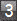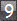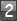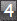Spirituality, New-Age - UFOs
Reverse engineering intense electromagnetic waves for antigravity

 Reverse engineering intense electromagnetic waves for antigravity propulsion of a space module from Extraterrestrial UFO technologies Reverse engineering intense electromagnetic waves for antigravity propulsion of a space module from Extraterrestrial UFO technologies India Daily Technology Team Analysis of flight pattern of reordered as well as simulated flight patterns of extraterrestrial UFOs is providing early clues to implement able antigravity propulsion systems. The Universe works with electromagnetic waves and acceleration and deceleration or change of intensity of theses waves known in Physics as electromagnetic flux. The gravity is just the application of the electromagnetic waves as well as flux in a definite direction. The antigravity propulsion can be obtained by applying an exactly opposite vector or tensor of order one. In ordinary three dimensional space, a vector has three components (contains three numbers, or three functions of position). In four dimensional space-time, a vector has four components. And, generally, in an n-dimensional space, a vector (tensor of order one) has n components. A vector may be thought of as an array of dimension one. This is because the components of a vector can be visualized as being written in a column or along a line, which is one dimensional. An example of a second order tensor is the so-called inertia matrix (or tensor) of an object. For three dimensional objects, it is a 3 x 3 = 9 element array that characterizes the behavior of a rotating body. As is well known to anyone who has played with a toy gyroscope, the response of a gyroscope to a force along a particular direction (described by a vector), is generally re-orientation along some other direction different from that of the applied force or torque. Thus, rotation must be characterized by a mathematical entity more complex than either a scalar or a vector; namely, a tensor of order two. There are yet more complex phenomena that require tensors of even higher order. For example, in Einstein's General Theory of Relativity, the curvature of space-time, which gives rise to gravity, is described by the so-called Riemann curvature tensor, which is a tensor of order four. Since it is defined in space-time, which is four dimensional, the Riemann curvature tensor can be represented as a four dimensional array (because the order of the tensor is four), with four components (because space-time is four dimensional) along each edge. That is, in this case, the Riemann curvature tensor has 4 x 4 x 4 x 4 = 256 components! [Fortunately, it turns out that only 20 of these components are mathematically independent of each other, vastly simplifying the solution of Einstein's equations]. The antigravity propulsion for a space craft with six degrees of freedom with no constraints requires tensor of order four. That will provide the very complex but amazing result of antigravity propulsion. Well the real complexity comes when you try to navigate such a spacecraft. The propulsion and navigation are complex but at least scientists have clear idea how to implement the same.

Reverse engineering intense electromagnetic waves for antigravity (Spirituality, New-Age - UFOs)    -    Author : John - USA3924 visitors since 2006-12-22
last update : 2006-12-22